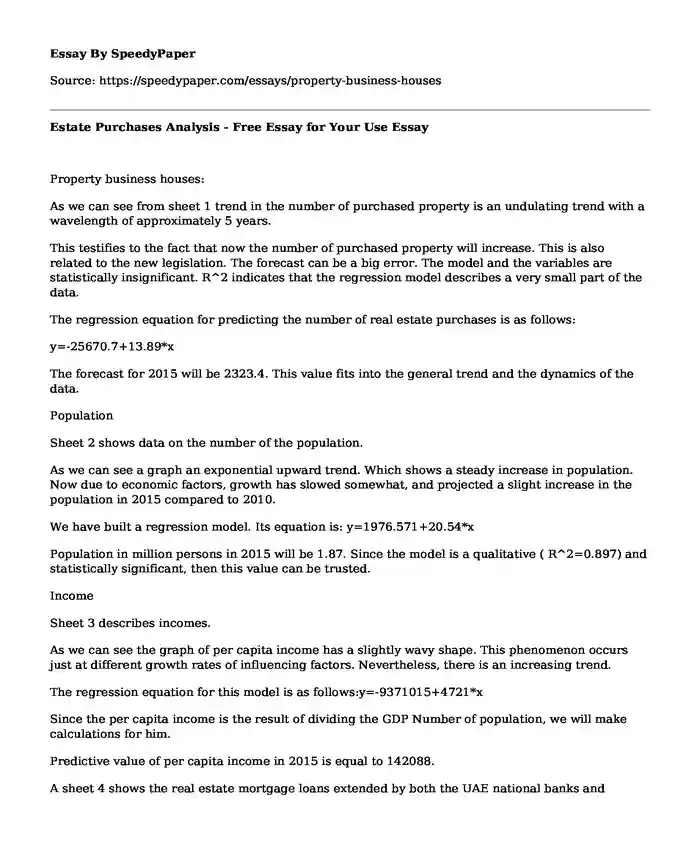# Estate Purchases Analysis - Free Essay for Your Use

Published: 2019-05-15Categories: Data analysis Statistics Pages: 3 Wordcount: 622 words
143 viewsIs your time best spent reading someone else’s essay? Get a 100% original essay FROM A CERTIFIED WRITER!

As we can see from sheet 1 trend in the number of purchased property is an undulating trend with a wavelength of approximately 5 years.

This testifies to the fact that now the number of purchased property will increase. This is also related to the new legislation. The forecast can be a big error. The model and the variables are statistically insignificant. R^2 indicates that the regression model describes a very small part of the data.

The regression equation for predicting the number of real estate purchases is as follows:

y=-25670.7+13.89*x

The forecast for 2015 will be 2323.4. This value fits into the general trend and the dynamics of the data.

Population

Sheet 2 shows data on the number of the population.

As we can see a graph an exponential upward trend. Which shows a steady increase in population. Now due to economic factors, growth has slowed somewhat, and projected a slight increase in the population in 2015 compared to 2010.

We have built a regression model. Its equation is: y=1976.571+20.54*x

Population in million persons in 2015 will be 1.87. Since the model is a qualitative ( R^2=0.897) and statistically significant, then this value can be trusted.

Income

Sheet 3 describes incomes.

As we can see the graph of per capita income has a slightly wavy shape. This phenomenon occurs just at different growth rates of influencing factors. Nevertheless, there is an increasing trend.

The regression equation for this model is as follows:y=-9371015+4721*x

Since the per capita income is the result of dividing the GDP Number of population, we will make calculations for him.

Predictive value of per capita income in 2015 is equal to 142088.

A sheet 4 shows the real estate mortgage loans extended by both the UAE national banks and foreign banks operating in UAE during 2006. Build a full regression model for such data is not possible.

The file shows two regression models, with which I would like to find the predicted value for 2015, but we are forced to question their adequacy.

Best fit model is: y=7854.867+3616*x

Predictive value is:33167.47.

Due to the fact that the data are intermittent, build a proper and good regression model, we can not. The model is inadequate. Although the graph we can see an increasing trend.

Sheet 5 shows Real estate mortgage loans (million AED).

These are permanent stable and slightly increasing character. The increase in interest will be due to increased incomes.

We built two regression models to predict separately Personal loan interest and Business loan interest.

The regression equation for the index of Interest, Personal loan is as follows: y=7.43+0.07*x

Forecast for 2015 is equal to 15.92

The regression equation for the index Business loan Interest, is as follows: y=11.47+0.03*x

Forecast for 2015 is equal to 14.67

Both models have a low quality, but fit into the overall trend. The models may be present large errors.

2. What will be the projected trends of prices of real estate in the housing sector?

(Use equation, Q= a + b P)

Based on the foregoing and the initial data we can draw conclusions. The greatest influence on the formation of real estate prices have dimensions of income and the price of land. As stated above, per capita incomes have undulating human character. This is due to periods of recession and growth in the economy, as well as uneven growth influencing factors (GDP and population). Unfortunately, it was never proved, and the price of land is not specified how much of the factors affect the pricing of supply and demand for real estate. This testifies to the incompleteness and lack of data for the formation of the equation Q= a + b P. Also, we do not have data on the cost to prior periods to form, for example regression model or extrapolation row.